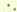## Resistance Of Beams

Before being able to calculate the strength of a beam the value of h must be found; for as the value of E in compression and tension is not the same, the neutral axis is now no longer fixed at the centre of gravity of section.

Assuming that Es/Ec= 10, and that the limiting stress of concrete and steel are simultaneously reached, and taking these values as c= 500 lbs. per square inch, and ft= 16,000 lbs. per square inch, then -

Maximum strain in compression maximum strain in tension = 500/Ec: 16,000/Es = 1 16,000/500x10 = 1:3.2.

Then from similar triangles (see Fig. 61) h d-h= 1 3.2.h/h +(d-h) =1/1+3.2.h =d/4.2.Safe resistance of concrete in compression =

2/3bh x 500 = 2/3 bd/4.2 x 500

For equilibrium the reinforcement must have equal tensile resistance.At 16,000 lbs. per square inch, necessary area of reinforcement = 2/3.bd/4.2.500 = 0.005bd.

16.000

That is to say, in order that both concrete and steel may simultaneously reach their limiting stress the reinforcement should have a sectional area of .5 per cent. of that of the concrete.

If wrought iron be used for the reinforcement, with a safe tensile strength of 11,000 lbs. per square inch; taking

Es/Ec10, as before, h:d - h = 1 11,000 = 1:2.2

500x10h=d/3.2

2/3.bd/3.2.500 and area of reinforcement = 11,000 = .0095 bd

= .95 per cent.

Thus if 1 per cent. or a larger proportion of reinforcement be used it matters little whether it consist of steel or wrought iron, and whichever can be obtained most cheaply may be employed.

It should be noticed that all concrete below the centre of the reinforcement has been left out of the calculation, for whatever thickness here exists the strength of the beam will not be affected if, as is usual, the resistance of the concrete in tension is neglected. Many formulae assume that this extra thickness of concrete is a particular fraction of the depth of the beam. For a beam 12 feet deep, one-sixth of depth may be a very suitable thickness, but this factor when applied to a beam 24 inches deep will be excessive.

Only the concrete above the centre of the reinforcement will therefore be considered, while a certain percentage of metal will be taken as indicating a percentage of the concrete above the reinforcement.

It will be seen in Fig. 54 that only a small portion of the concrete is of use in resisting compression, and it does not follow, although the greatest use is made of the steel, that this proportion of 1/2 per cent. will produce the cheapest and most convenient arrangement.Fig. 54.

The effect of employing a reinforcement of 1 per cent. will now be investigated.

Let a = area of reinforcement.

Then resistance of concrete in compression = resistance of steel in tension.

2/3 bhc = aft; but a = bd/100 , and c = 500.2x500 /3bh= bd /100ft.ft = 100,000h.

3d h:d-h=1:100,000h = 1: 20h. (A)3dx500x10 3d.h = d =.319d

1+20h

3d

Substituting this value in (A) ft=100,000x.319d =10,633 lbs. per square inch. 3d The moment of resistance -

= 2/3 bhc(d-2/5h)(seeFig. 54 = 2/3 bhcx.87d. = 92.77 bd2. To arrive at a general formula, let a = sectional area of reinforcement, and r=Es=Ec ft=2bhc

Then 2/3 bhc = aft.3a h:d-h=1: 2bhc3axcxr h = d 1+2bh3ar or if p = percentage of reinforcement, a=bdp/100, and substituting this value in the above formula,and the moment of resistance,

Mr =2/3bhc (d-2/5h). The following table gives information found by the above formulae for various percentages of reinforcement, which it should be noticed are percentages upon the area of concrete above the level of the centre of the reinforcement, the concrete below this being neglected.

Es/E = 10. Limiting stress on concrete = 500 lbs.

 Percentage of Reinforcement upon Concrete above Level of Do. p Distance ofNeutral Axis from Surface at CompressionSide of Beam.h Tensile Stress on Reinforcement when c=500.ft Moment ofResistance. 0.50 0.238 d 16,000 lbs. 71.8 bd2 0.75 0.284 ,, 12,622 ,, 83.9 ,, 1.00 0.319 ,, 10,633 ,, 92.8 ,, 1.25 0.349 ,, 9.307 ,, 100.1 ,, 1.50 0.375 ,, 8,333 ,, 106.2 ,, 2.00 0.418 ,, 6,966 ,, 116.0 ,, 2.50 0.453 ,, 6,040 ,, 123.6 ,, 3.00 0.482 ,, 5,356 ,, 129.7 ,, 3.50 0.508 ,, 4.838 ,, 134.9 ,, 4.00 0.53l ,, 4.425 ,, 139.4 ,,

In Fig. 55 are shown the sections of four beams with varying percentages of reinforcement, all being calculated to give the same moment of resistance of 360,000 inch-pounds. For the sake of comparison their widths have been kept constant. It will be seen that very little saving in depth is obtained by the use of a higher percentage of reinforcement than 2 per cent. For simple beams of this description a reinforcement of 1 per cent. is very suitable, and has been largely adopted; while for tee beams, 1/2 per cent. on area B x D may be adhered to (see Fig. 57).

## Slabs

A floor slab is usually supported by beams along its four edges. The slab thus supported may be considered as acting as a beam in two directions at right angles to one another. If the slab be square, the load will be evenly distributed among the four edges, and the maximum bending moment and shear will consequently be only half such as would occur were the slab supported on two edges only. Thus with a square slab BM =wl2/16, or if the slab be continuous, and the reinforcement be transferred to the upper surface in passing over the supports, the BM at centre may be taken as wl2/24, and over supports, say wl2/20. When a slab is twice as long as it is broad the supports at either end only very slightly reduce the bending moment at the centre, and the slab may then be considered as a simple beam supported along its two long sides.